# Seaborn | Distribution Plots

Seaborn is a Python data visualization library based on Matplotlib. It provides a high-level interface for drawing attractive and informative statistical graphics. This article deals with the distribution plots in seaborn which is used for examining univariate and bivariate distributions. In this article we will be discussing 4 types of distribution plots namely:

1. joinplot
2. distplot
3. pairplot
4. rugplot

Besides providing different kinds of visualization plots, seaborn also contains some built-in datasets. We will be using the tips dataset in this article. The “tips” dataset contains information about people who probably had food at a restaurant and whether or not they left a tip, their age, gender and so on. Lets have a look at it.

Code :

 `# import thr necessary libraries ` `import` `seaborn as sns ` `import` `matplotlib.pyplot as plt ``%` `matplotlib inline ` ` `  `# to ignore the warnings  ` `from` `warnings ``import` `filterwarnings ` ` `  `# load the dataset ` `df ``=` `sns.load_dataset(``'tips'``) ` ` `  `# the first five entries of the dataset ` `df.head() `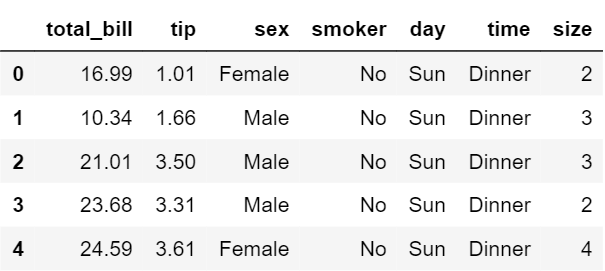Now, lets proceed onto the plots.

## Displot

It is used basically for univariant set of observations and visualizes it through a histogram i.e. only one observation and hence we choose one particular column of the dataset.
Syntax:

`distplot(a[, bins, hist, kde, rug, fit, ...])`

Example:

 `# set the background style of the plot ` `sns.set_style(``'whitegrid'``) ` `sns.distplot(df[``'total_bill'``], kde ``=` `False``, color ``=``'red'``, bins ``=` `30``) `

Output: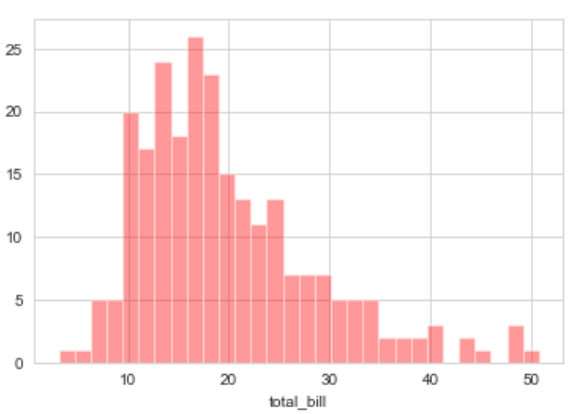Explanation:

• KDE stands for Kernel Density Estimation and that is another kind of the plot in seaborn.
• bins is used to set the number of bins you want in your plot and it actually depends on your dataset.
• color is used to specify the color of the plot

Now looking at this we can say that most of the total bill given lies between 10 and 20.

## Joinplot

It is used to draw a plot of two variables with bivariate and univariate graphs. It basically combines two different plots.
Syntax:

`jointplot(x, y[, data, kind, stat_func, ...])    `

Example:

 `sns.jointplot(x ``=``'total_bill'``, y ``=``'tip'``, data ``=` `df) `

Output: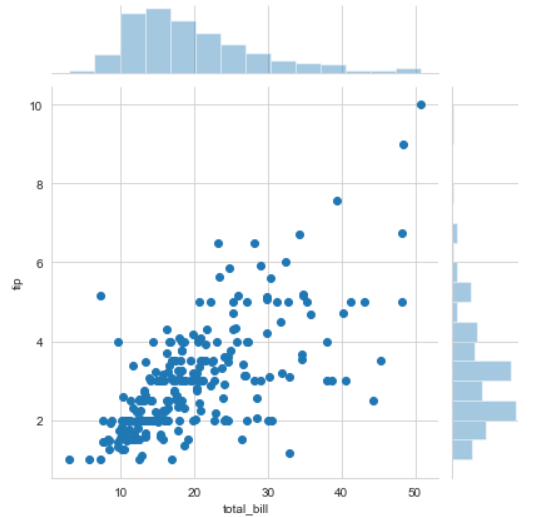`sns.jointplot(x ``=``'total_bill'``, y ``=``'tip'``, data ``=` `df, kind ``=``'kde'``) ` `# KDE shows the density where the points match up the most `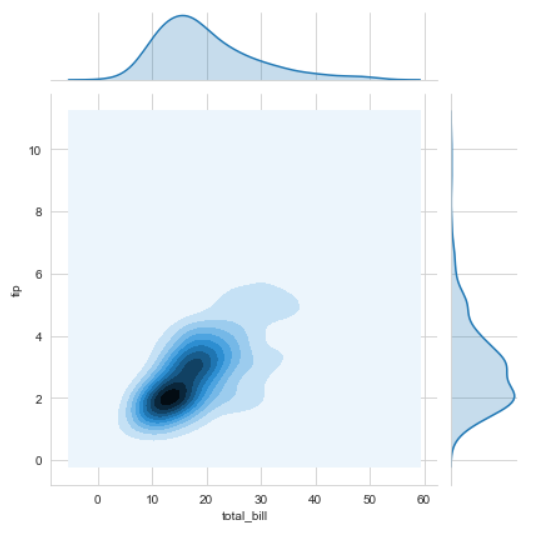Explanation:

• kind is a variable that helps us play around with the fact as to how do you want to visualise the data.It helps to see whats going inside the joinplot. The default is scatter and can be hex, reg(regression) or kde.
• x and y are two strings that are the column names and the data that column contains is used by specifying the data parameter.
• here we can see tips on the y axis and total bill on the x axis as well as a linear relationship between the two that suggests that the total bill increases with the tips.
•

## Pairplot

It represents pairwise relation across the entire dataframe and supports an additional argument called hue for categorical separation. What it does basically is create a jointplot between every possible numerical column and takes a while if the dataframe is really huge.

Syntax:

`pairplot(data[, hue, hue_order, palette, …])    `

Example:

 `sns.pairplot(df, hue ``=``"sex"``, palette ``=``'coolwarm'``) `

Output: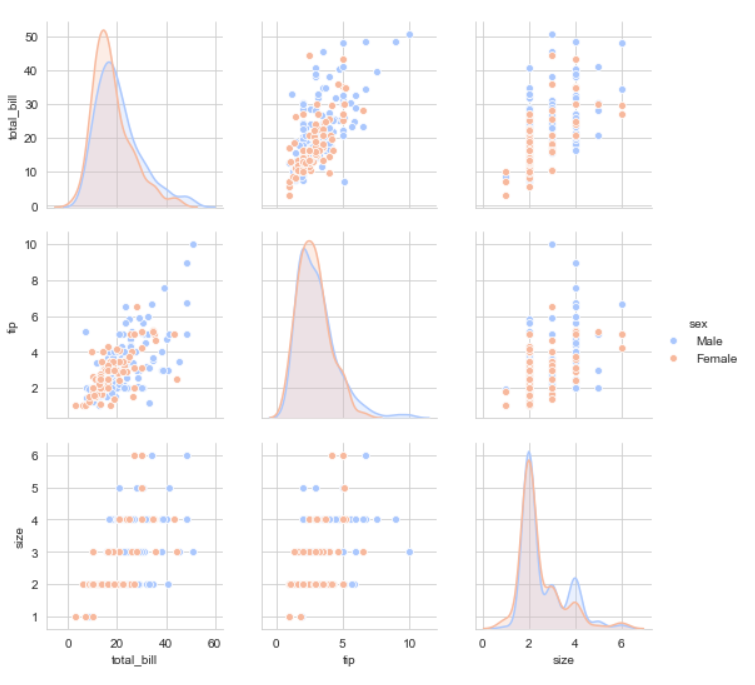Explanation:

• hue sets up the categorical separation between the entries if the dataset.
• palette is used for designing the plots.

## Rugplot

It plots datapoints in an array as sticks on an axis.Just like a distplot it takes a single column. Instead of drawing a histogram it creates dashes all across the plot. If you compare it with the joinplot you can see that what a jointplot does is that it counts the dashes and shows it as bins.

Syntax:

`rugplot(a[, height, axis, ax])  `

Example:

 `sns.rugplot(df[``'total_bill'``]) `

Output: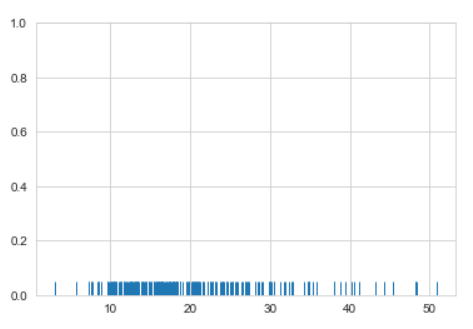My Personal Notes arrow_drop_upCheck out this Author's contributed articles.

If you like GeeksforGeeks and would like to contribute, you can also write an article using contribute.geeksforgeeks.org or mail your article to contribute@geeksforgeeks.org. See your article appearing on the GeeksforGeeks main page and help other Geeks.

Please Improve this article if you find anything incorrect by clicking on the "Improve Article" button below.

Article Tags :
Practice Tags :

1

Please write to us at contribute@geeksforgeeks.org to report any issue with the above content.